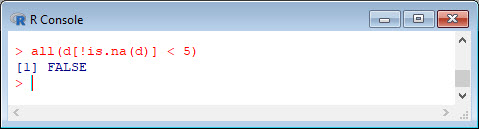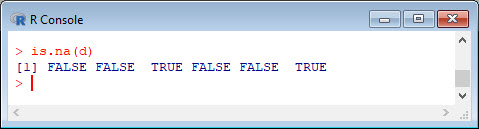# Quick start with R: Using any() and all() commands (Part 18)

##### Test for the existence of particular values using the `any()` and `all()` commands

Create a vector `b`:
```b <- c(7, 2, 4, 3, -1, -2, 3, 3, 6, 8, 12, 7, 3) b```
Use the function `any()` to see if there is any element in `b` equal -4 and less than 5:
```any(b == -4) any(b < 5)```Both commands work on logical vectors. Use `any()` to check for missing data in a vector or an array.
```d <- c(3, 2, NA, 5, 6, NA) d any(is.na(d))```Of course, we can check for non-missing data too.
`any(!is.na(d))`The `any()` command is helpful when checking for particular values in large data sets.
You can use the `all()` command to check whether all elements in a given vector or array satisfy a particular condition. For example, let’s see whether all non-missing values in `d` are less than 5. Here we note noting that the command `is.na()` identifies missing data and that the syntax `!is.na()` identifies non-missing data.
`all(d[!is.na(d)] < 5)`Now check whether all non-missing elements are less than 7.
`all(d[!is.na(d)] < 7)`The syntax above looks formidable. However, `is.na()` identifies missing elements by creating a logical vector whose elements are either `TRUE` or `FALSE`.
`is.na(d)`The syntax `!is.na(d)` gives the opposite logical vector and counts non-missing data. Then, `d[!is.na(d)]` gives the elements of `d` that are-non missing. Finally, we apply the `all()` command, and include the condition that all elements are less than 7.
That wasn’t so hard! In Blog 19 I will present another tip for data analysis in R.
See you later!
David

#### Annex: R codes used

```# Create a vector b.
b <- c(7, 2, 4, 3, -1, -2, 3, 3, 6, 8, 12, 7, 3)
b

# Check if there is any element in b equal -4 and less than 5:
any(b == -4)
any(b < 5)

# Check for missing data in a vector d.
d <- c(3, 2, NA, 5, 6, NA)
d
any(is.na(d))

# Check for non-missing data.
any(!is.na(d))

# Check if all non-missing values in d are less than 5.
all(d[!is.na(d)] < 5)

# Check if all non-missing values in d are less than 7.
all(d[!is.na(d)] < 7)

# Identify missing elements in d.
is.na(d)
```